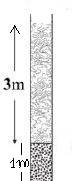# Pressure at the bottom of a container containing two liquids

Akash47

## Homework Statement(In the attachment)There is two layers of liquids in the container .The density of the upper layer of the container, ##ρ_1=800kg/m^3## and the density of the bottom layer of the container ,##ρ_2=13600kg/m^3## .What is the pressure at the bottom of the container?

## Homework Equations

Pressure at height ##h## in a liquid from the surface, ##p=hρg##

## The Attempt at a Solution

I have first calculated the pressure at the bottom of the first layer,##p_1=(3*800*9.8)=23520~pa##.Then taking the bottom of the first layer as the surface of the second layer,pressure at the bottom of the second layer,##p_2=(1*13600*9.8)~pa=133280~pa##.So the total pressure at the bottom of the container,##p=p_o+p_1+p_2=(101325+23520+133280)~pa=258125~pa.##Is my solution correct?If yes,then how can I make the solution more better(as I think it's not enough).If my solution is wrong,then tell me what's wrong and give hint for the solution.

## Answers and Replies

Homework Helper
Gold Member
how can I make the solution more better
Some data you are using is only to two significant figures, so you should claim no greater precision in your answer. I would round it to 258000 Pa, or maybe even 260000.

Akash47
I've made an assumption of adding the pressures at the bottom of different layers of liquids.I am looking for a reasonable logic for that.Is my solution overall correct?

Homework Helper
Gold Member
I've made an assumption of adding the pressures at the bottom of different layers of liquids.I am looking for a reasonable logic for that.Is my solution overall correct?
Yes, of course. It is a simple balance of forces. The normal force from the bottom of the container must balance the sum of the downward forces on the lower liquid: gravity and the normal force from the upper liquid. Likewise for the upper liquid.

Akash47
Yes, of course. It is a simple balance of forces. The normal force from the bottom of the container must balance the sum of the downward forces on the lower liquid: gravity and the normal force from the upper liquid. Likewise for the upper liquid.
I haven't understand your language properly as I am not fluent in English.Please express your words more easily.

Homework Helper
Gold Member
I haven't understand your language properly as I am not fluent in English.Please express your words more easily.
Consider the two volumes of liquid as objects with forces operating on them.
The upper volume has the force Fa of the atmosphere pressing down on top, a normal force Fm pushing up from the lower volume, and gravity Fgu. These three forces are in balance, so Fm=Fa+Fgu.
The lower volume likewise has three forces, Fb=Fm+Fgl, where Fb is the normal force from the bottom of the tube and Fgl is the force of gravity on the lower volume.
Combining these we have Fb=Fa+Fgu+Fgl.
So the pressures add up as you assumed.

•Akash47
Akash47
Thankss....